Next: Flow in Slowly-Varying Channels Up: Incompressible Viscous Flow Previous: Poiseuille Flow

# Taylor-Couette Flow

Consider two thin cylindrical shells with the same vertical axis. Let the inner and outer shells be of radius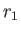and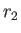, respectively. Suppose that the annular region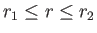is filled with fluid of density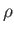and viscosity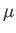. Let the inner and outer cylinders rotate at the constant angular velocities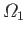and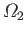, respectively. We wish to determine the steady flow pattern set up within the fluid. Incidentally, this type of flow is generally known as Taylor-Couette flow, after Maurice Couette and Geoffrey Taylor (1886-1975).

It is convenient to adopt cylindrical coordinates,,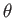,, whose symmetry axis coincides with the common axis of the two shells. Thus, the inner and outer shells correspond to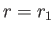and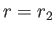, respectively. Suppose that the flow velocity within the fluid is written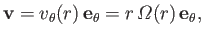(10.30)

where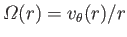is the angular velocity profile. Application of the no slip condition at the two shells leads to the boundary conditions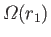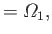(10.31)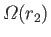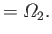(10.32)

It again follows from Section 1.19 that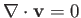and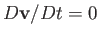. Hence, Equation (10.2) reduces to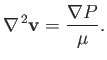(10.33)

Assuming that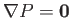within the fluid, because any flow is driven by the angular rotation of the two shells, rather than by pressure gradients or gravity, and again making use of the results quoted in Section 1.19, the previous expression yields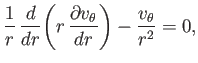(10.34)

or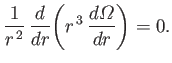(10.35)

The solution of Equation (10.35) that satisfies the boundary conditions is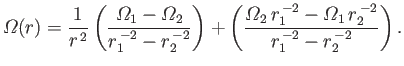(10.36)

It can be seen that this angular velocity profile is a combination of the solid body rotation profile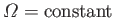, and the irrotational rotation profile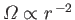.

From Section 1.19, the only non-zero component of the viscous stress tensor within the fluid is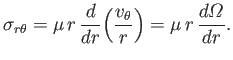(10.37)

Thus, the viscous torque (acting in the-direction) per unit height (in the-direction) exerted on the inner cylinder is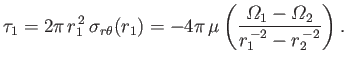(10.38)

Likewise, the torque per unit height exerted on the outer cylinder is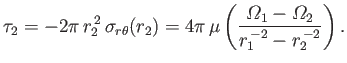(10.39)

As expected, these two torques are equal and opposite, and act to make the two cylinders rotate at the same angular velocity (in which case, the fluid between them rotates as a solid body).Next: Flow in Slowly-Varying Channels Up: Incompressible Viscous Flow Previous: Poiseuille Flow
Richard Fitzpatrick 2016-01-22